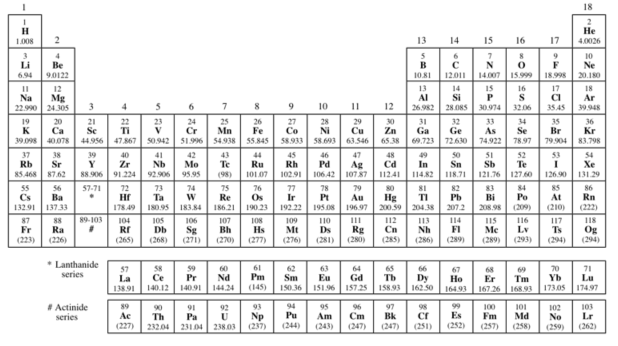Problem: U-235 decays in the following series of steps: alpha decay, beta decay, alpha decay, beta decay, alpha decay, alpha decay, alpha decay, alpha decay, beta decay, alpha decay, beta decay. After these 11 decays, the final daughter nuclei are stable. What is the final daughter nucleus? A periodic table has been attached to this problem to help.

87% (48 ratings)
Problem Details

U-235 decays in the following series of steps: alpha decay, beta decay, alpha decay, beta decay, alpha decay, alpha decay, alpha decay, alpha decay, beta decay, alpha decay, beta decay. After these 11 decays, the final daughter nuclei are stable. What is the final daughter nucleus? A periodic table has been attached to this problem to help.